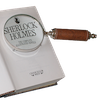#### You may also like### Number Detective

Follow the clues to find the mystery number.### Six Is the Sum

What do the digits in the number fifteen add up to? How many other numbers have digits with the same total but no zeros?### ABC

In the multiplication calculation, some of the digits have been replaced by letters and others by asterisks. Can you reconstruct the original multiplication?

# The Thousands Game

##### Age 7 to 11Challenge Level

You had to think very carefully to answer this question. Amy from St Albans Primary School, Kevin from CIS, Hong Kong, Kirsty who goes to Steeple Morden and Ruth all reasoned it through very clearly. Here is Amy's argument:

When Jill did it, she picked out $7, 8, 6$ and $3$. To make the biggest even number she got $8736$. Vincent picked out $4, 2, 9$ and $1$. The biggest even number is $9412$. Vincent won because he had the highest even number. I worked this out because $9000$ is bigger than $8000$.
Next it was the highest odd number. Belinda picked out $4, 0, 6$ and $9$. The highest odd number from these is $6409$. Ali picked out $5, 2, 1$ and $7$. His highest odd number is $7521$. Ali's number is higher than Belinda's because $7000$ is higher than $6000$.
Next is the lowest even number. Ben picked out $7, 0, 3$ and $9$ and the lowest even number is $3790$. Rohan picked $8, 1, 5$ and $4$ and his number is $1458$. Rohan won because $1000$ is lower than $3000$.
Alice got $7, 8, 4$ and $9$ and Chloe got $1, 0, 3$ and $5$. The numbers they got which are nearest to $5000$ are $4987$ and $5013$. I used an abacus to show that the difference is $13$ for both and so it is a draw.

Thank you for a very clear solution, Amy.